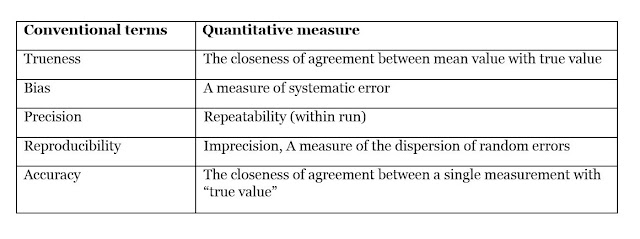### Assay Precision & Accuracy

Trueness and Accuracy
The trueness of measurement can be defined as the closeness of agreement of between the average value obtained from large series of results of measurement and a true (actual) value. The difference between the average value and the true value is called the bias, which is inversely related to the trueness. Trueness can be evaluated by comparison of measurements by given method and a reference method. Such an evaluation may be carried out by parallel measurements of a set of test samples or by measurement of reference materials. The ISO has introduced the trueness expression as a replacement for the term “accuracy”. Accuracy is the closeness of the agreement between the result of measurement and a true concentration of the analyte. Accuracy is thus influenced by both bias and imprecision and in this way reflects the total error.

Precision
Precision may be defined as the clones of agreement between independent results of measurement obtained under specified conditions. The degree of precision is usually expressed on the basis of statistical measures of impression such as the SD or CV( CV= SD/mean), which thus is inversely related to precision. The imprecision of measurement is to random error and it is independent of trueness (accuracy) of measurements. Precision often depends on the concentration of the analyte being considered.

Repeatability:
The closeness of agreement between results of successive measurements carried out under the same condition (i.e. corresponding to within-run precision).

Reproducibility:
The closeness of agreement between results of measurement performed under a changed condition of measurements (e.g. time, operator, calibrations and reagent lots). The precision can be used as intra-assay (within-run) and inter-assay (between-run) precision. To estimate the variation or imprecision, a common approach is to measure duplicate samples in a series of runs. And the estimate of the imprecision improves the more observations are available.Model of Precision and Accuracy. Precision and Accuracy are independent parameters for given assay